Test: Vector Analysis - 1

# Test: Vector Analysis - 1

Test Description

## 10 Questions MCQ Test Topicwise Question Bank for Electrical Engineering | Test: Vector Analysis - 1

Test: Vector Analysis - 1 for Electrical Engineering (EE) 2023 is part of Topicwise Question Bank for Electrical Engineering preparation. The Test: Vector Analysis - 1 questions and answers have been prepared according to the Electrical Engineering (EE) exam syllabus.The Test: Vector Analysis - 1 MCQs are made for Electrical Engineering (EE) 2023 Exam. Find important definitions, questions, notes, meanings, examples, exercises, MCQs and online tests for Test: Vector Analysis - 1 below.
Solutions of Test: Vector Analysis - 1 questions in English are available as part of our Topicwise Question Bank for Electrical Engineering for Electrical Engineering (EE) & Test: Vector Analysis - 1 solutions in Hindi for Topicwise Question Bank for Electrical Engineering course. Download more important topics, notes, lectures and mock test series for Electrical Engineering (EE) Exam by signing up for free. Attempt Test: Vector Analysis - 1 | 10 questions in 30 minutes | Mock test for Electrical Engineering (EE) preparation | Free important questions MCQ to study Topicwise Question Bank for Electrical Engineering for Electrical Engineering (EE) Exam | Download free PDF with solutions
 1 Crore+ students have signed up on EduRev. Have you?
Test: Vector Analysis - 1 - Question 1

### If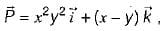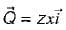φ = xy2z3 then match List-I with List-II and select the correct answer using the codes given below the lists: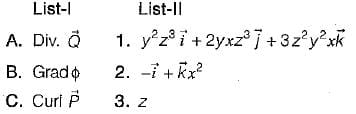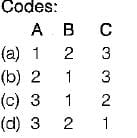Detailed Solution for Test: Vector Analysis - 1 - Question 1

Here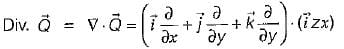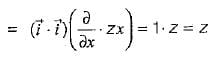Also,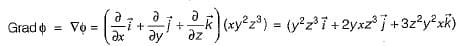And,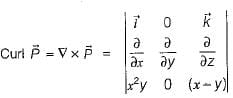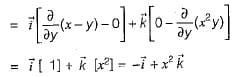Test: Vector Analysis - 1 - Question 2

### The unit vector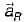which points from z = h on the z-axis towards (r, φ, 0) in cylindrical co-ordinates as shown below is given by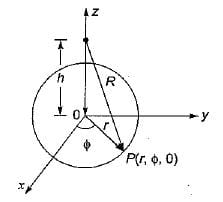Detailed Solution for Test: Vector Analysis - 1 - Question 2

Let the unit vector be given by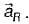Now,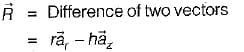∴ Unit vector,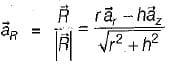Test: Vector Analysis - 1 - Question 3

### If the vector V given below is irrotational, then the values of a, b and c will be respectively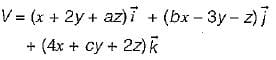Detailed Solution for Test: Vector Analysis - 1 - Question 3

Since the given vector is irrotational, therefore curl V = 0 or,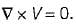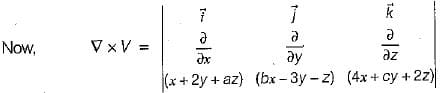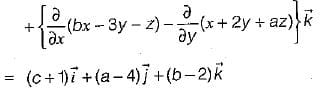Since,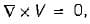therefore, a = 4, b = 2, and c = -1

Test: Vector Analysis - 1 - Question 4

Match List-I (Vector Identities) with List-ll. (Equivalent expression) and select the correct answer using the codes given below the lists: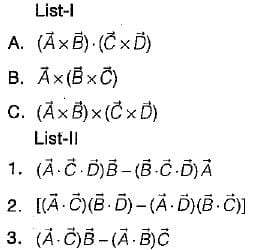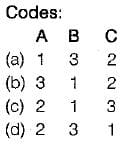Detailed Solution for Test: Vector Analysis - 1 - Question 4
•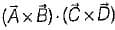is called “ product of four vectors ”.
•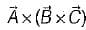is called “vector triple product”.
•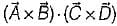is called "vector product of four vectors ”.
Test: Vector Analysis - 1 - Question 5

The vector differential operator,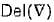in spherical co-ordinate system is given by

Test: Vector Analysis - 1 - Question 6

Assertion (A): Divergence of a vector function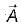at each point gives the rate per unit volume at which the physical entity is issuing from that point.

Reason (R): If some physical entity is generated or absorbed within a certain region of the field, then that region is known as source or sink respectively and if there are no sources or sinks in the field, the net outflow of the incompressible physical entity over any part of the region is zero. However, the net outflow is said to be positive, if the total strength of the sources are greater than the total strength of sink and vice-versa.

Detailed Solution for Test: Vector Analysis - 1 - Question 6

Both assertion and reason are true and reason is the correct explanation of assertion. Reason is the physical interpretation of divergence.

Test: Vector Analysis - 1 - Question 7

Which of the following identity is not true?

Detailed Solution for Test: Vector Analysis - 1 - Question 7
•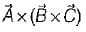is called “vector triple product" which is a correct expression.
•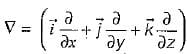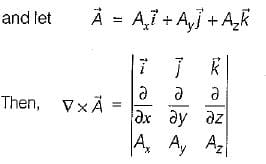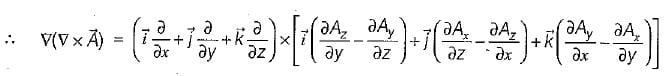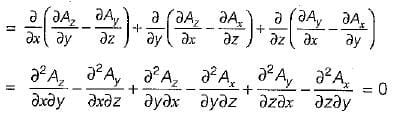•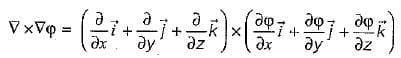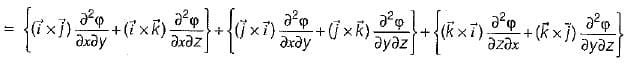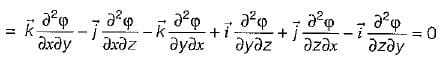Test: Vector Analysis - 1 - Question 8

The vector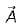directed from (2, - 4,1) to (0, -2,0) in Cartesian coordinates is given by

Detailed Solution for Test: Vector Analysis - 1 - Question 8

The vector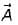is given as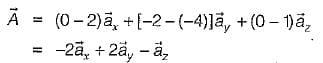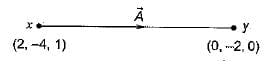Test: Vector Analysis - 1 - Question 9

What is the value of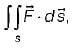where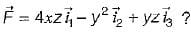Here, s is the surface bounded by x = 0, x = 1, y = o, y = 1, z = 0, z =1 and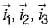are unit vectors along x, yand z axes respectively.

Detailed Solution for Test: Vector Analysis - 1 - Question 9

By divergence theorem,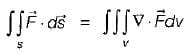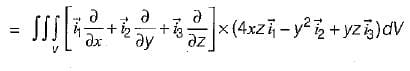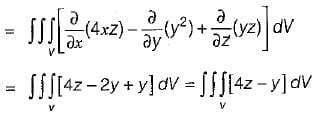Since, the surface s is bounded by x = 0, 1; y = 0, 1 and z = 0, 1 so, putting the limits, we have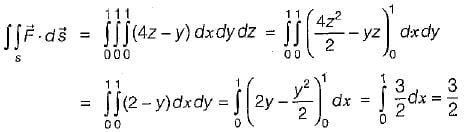Test: Vector Analysis - 1 - Question 10

The vector field given by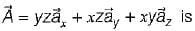Detailed Solution for Test: Vector Analysis - 1 - Question 10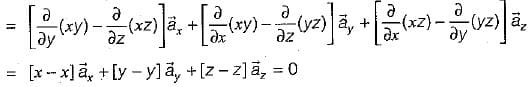The vector field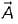will be irrotational,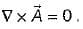Now,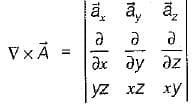Hence,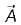is irrotationai.
The vector field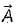will be solenoidal,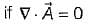Here,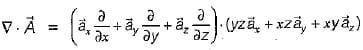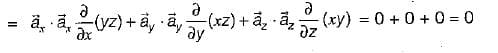Hence,is solenoidal.

## Topicwise Question Bank for Electrical Engineering

207 tests
 Use Code STAYHOME200 and get INR 200 additional OFF Use Coupon Code
Information about Test: Vector Analysis - 1 Page
In this test you can find the Exam questions for Test: Vector Analysis - 1 solved & explained in the simplest way possible. Besides giving Questions and answers for Test: Vector Analysis - 1, EduRev gives you an ample number of Online tests for practice

207 tests